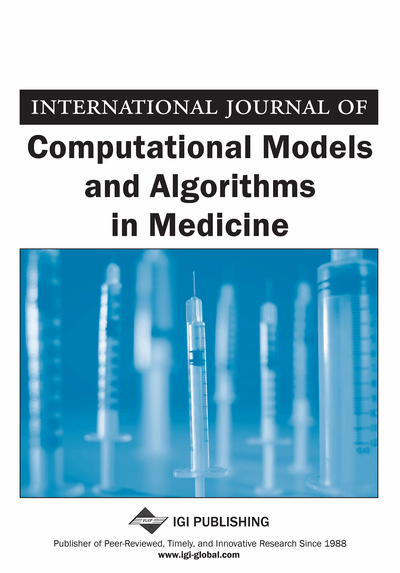# A New Mechanical Algorithm for Calculating the Amplitude Equation of the Reaction-Diffusion Systems

Houye Liu (Wenzhou University, Wenzhou, China) and Weiming Wang (Wenzhou University, Wenzhou, China)
DOI: 10.4018/ijcmam.2012100103
Available
\$37.50
No Current Special Offers

## Abstract

Amplitude equation may be used to study pattern formatio. In this article, the authors establish a new mechanical algorithm AE_Hopf for calculating the amplitude equation near Hopf bifurcation based on the method of normal form approach in Maple. The normal form approach needs a large number of variables and intricate calculations. As a result, deriving the amplitude equation from diffusion-reaction is a difficult task. Making use of our mechanical algorithm, we derived the amplitude equations from several biology and physics models. The results indicate that the algorithm is easy to apply and effective. This algorithm may be useful for learning the dynamics of pattern formation of reaction-diffusion systems in future studies.
Article Preview
Top

## Introduction

Turing (1952) showed that under certain conditions, reaction and diffusion processes alone could lead to the symmetry-breaking instability (i.e., Turing instability) of a system (i.e., Turing system) from a homogeneous state to a stationary patterned state (Setayeshgar & Cross, 1999). Turing system can generate stationary patterns such as stripes and/or spots. Turing instability mechanism has particular relevance to pattern formation in nonlinear complex systems. Nowadays, pattern formation is one of the central problems of the natural, social, and technological science (Medvinsky et al., 2002). Especially in population dynamics, pattern can clarify the distribution and development of different species. There are a number of theoretical and numerical studies of pattern formation by using weakly nonlinear analysis (Chen & Vinals, 1999; Dufiet & Boissonade, 1996; Ipsen et al., 1998; Ipsen et al., 2000; Malomed, 1993; Pena & Perez-Garca, 2000). Among these studies, the authors have focused on pattern formation from a spatially uniform state that is near the transition from linear stability to linear instability by using the standard bifurcation-theoretic tools such as amplitude equations (Pearson, 1993). The amplitude equation formalism is a natural scheme to extract universal properties of pattern formation (Gunaratne et al., 1994). Briefly, the idea of an amplitude equation for an oscillatory reaction-diffusion system is to represent the state of the system through a complex amplitude (real amplitude and phase) which varies much more slowly in space and time than the original dynamical variables (Ipsen et al., 1998).

Near supercritical Hopf bifurcation, the amplitude equation of reaction-diffusion system can be reduced to the complex Ginzburg-Landau equation (CGLE). CGLE describes the time evolution of the amplitude of the oscillatory system, shows rich behavior and has been applied in a variety of contexts (Leppanen, 2004). The CGLE, i.e., amplitude equation, takes the form:

(1) where a is the complex amplitude of the oscillation. I is the imaginary unit, and ϖ, α, β are adjustable parameters.

Some valid methods for obtaining the coefficients of the amplitude equation have been developed in recent years, such as multi-scale expansion (Newell, et al., 1993), center manifold reduction (Callahan & Knobloch, 1999), etc. Besides, there is another method called normal form approach presented by Ispen et al. (1998). This method can derive normal forms and related amplitude equations for flows and discrete dynamics on the center manifold of a dynamical system at local bifurcations.

However, the normal form approach method requires a huge number of calculations. The objective of this chapter is to establish a promising mechanical algorithm in Maple for calculating the amplitude equation near Hopf bifurcation.

Top

## Basic Methods

Let us first recall the basic principles of the method of normal form approach for calculating the coefficients of amplitude equation. For more details, we refer to (Ipsen et al., 1998; Ipsen et al., 2000). Here we pay attention to the following reaction-diffusion system:

(2) where u and v stand for the density of two species, μ the bifurcation parameter, f and g nonlinear function, and d1, d2 the diffusion coefficients.is the usual Laplacian operator in two-dimensional space. To simplify, system (2) can be rewritten as:(3) where V is a vector (V ϵ R2), and μ is a set of parameters (μ ϵ R5). The stationary point of system (2) is Vs(μ). D = diag(d1, d2) is the diffusive matrix. And J, the so-called Jacobian matrix at the stationary point Vs(μ), yields:

## Complete Article List

Search this Journal:
Reset
Volume 4: 2 Issues (2014)
Volume 3: 4 Issues (2012)
Volume 2: 4 Issues (2011)
Volume 1: 4 Issues (2010)
View Complete Journal Contents Listing